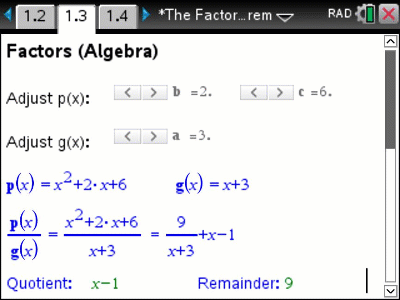# Activities

•• ##### AuthorAust Senior

50 Minutes

• ##### Device
•TI-Nspire™ CX CAS
• ##### Software

TI-Nspire™ CAS

4.5

## Factor and Remainder#### Activity Overview

Division of numerical quantities is a great way to start exploring and understanding division of polynomials. In this activity students start with what they know about division of numerical quantities and then apply this to division of polynomials. This assists with some of the terminology and also developing a deeper understanding. Polynomials can be regenerated dynamically and rapidly including the resultant quotient and, where applicable, the remainder, this helps students identify and explain patterns. The visual representation of polynomial division also helps students understand the factor and remainder theorem, particularly expressing a polynomial as a product of its linear factors.

#### Objectives

• Understand the factor theorem
• Understand the remainder theorem
• Develop algebraic expectation pertaining to outcomes of polynomial division

• Quotient
• Remainder
• Divisor
• Factor
• Remainder
• Polynomial
• Degree